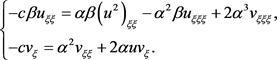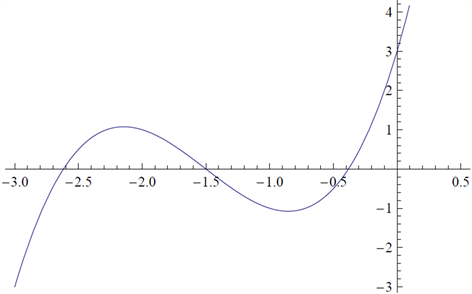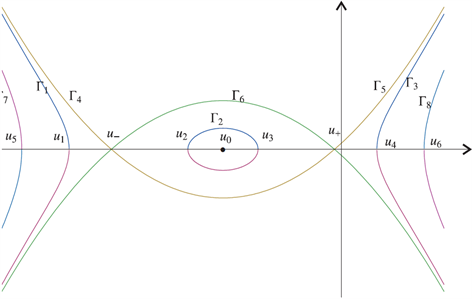# Boiti-Leon-Pempinelle方程新的行波解New Traveling Wave Solutions for Boiti-Leon-Pempinelle Equation

• 全文下载: PDF(514KB)    PP.1537-1542   DOI: 10.12677/AAM.2018.712179
• 下载量: 369  浏览量: 454

This paper is concerned with smooth and non-smooth traveling wave solutions of the Boiti-Leon- Pempinelle equation based on the bifurcation method of dynamical systems. First, we establish a new Hamiltonian function on the variable u(x, t). Second, we prove that the corresponding traveling wave system of the Boiti-Leon-Pempinelle equation exists new traveling wave solutions. Our work extends some previous results.

1. 引言

$\left\{\begin{array}{l}{u}_{yt}={\left({u}^{2}\right)}_{xy}-{u}_{xxy}+2{v}_{xxx},\\ {v}_{t}={v}_{xx}+2u{v}_{x},\end{array}$ (1.1)

$u\left(x,y,t\right)=u\left(\xi \right),\text{\hspace{0.17em}}\text{\hspace{0.17em}}\text{\hspace{0.17em}}v\left(x,y,t\right)=v\left(\xi \right),\text{\hspace{0.17em}}\text{\hspace{0.17em}}\text{\hspace{0.17em}}\xi =\alpha x+\beta y-ct,$ (1.2)(1.3)

$-c\beta u=\alpha \beta {u}^{2}-{\alpha }^{2}\beta {u}_{\xi }+2{\alpha }^{3}{v}_{\xi }+{g}_{1}\xi +g.$ (1.4)

${g}_{1}=0$ ，则(1.4)可简化为

${v}_{\xi }=\frac{1}{2{\alpha }^{3}}\left(-c\beta u-\alpha \beta {u}^{2}+{\alpha }^{2}\beta {u}_{\xi }-g\right).$ (1.5)

$u=-\frac{1}{2\alpha }\left(c+{\alpha }^{2}\frac{{v}_{\xi \xi }}{{v}_{\xi }}\right).$ (1.6)

${v}_{\xi \xi }=\frac{1}{2{\alpha }^{3}}\left(-c\beta {u}_{\xi }-2\alpha \beta u{u}_{\xi }+{\alpha }^{2}\beta {u}_{\xi \xi }\right).$ (1.7)

${\alpha }^{4}\beta {u}_{\xi \xi }-{\alpha }^{2}{g}_{1}-\left(2\alpha u+c\right)\left(c\beta u+\alpha \beta {u}^{2}+g\right)=0.$ (1.8)

$\left\{\begin{array}{l}\frac{\text{d}u}{\text{d}\xi }=y,\\ \frac{\text{d}y}{\text{d}\xi }=\frac{2}{{\alpha }^{2}}{u}^{3}+\frac{3c}{{\alpha }^{3}}{u}^{2}+\frac{1}{{\alpha }^{4}\beta }\left({c}^{2}\beta +2\alpha g\right)u+\frac{cg}{{\alpha }^{4}\beta },\end{array}$ (1.9)

$H\left(u,y\right)=\frac{1}{2}{y}^{2}-\frac{1}{2{\alpha }^{2}}{u}^{4}-\frac{c}{{\alpha }^{3}}{u}^{3}-\frac{1}{2{\alpha }^{4}\beta }\left({c}^{2}\beta +2\alpha g\right){u}^{2}-\frac{cg}{{\alpha }^{4}\beta }u=h,$ (1.10)

1) 本文在函数 $u\left(x,t\right)$ 之上建立了系统(1.1)一个新的哈密顿函数。

2) 基于定性理论和哈密顿函数，我们证明了该系统异宿轨的一个有趣现象，即异宿轨的存在与积分常数g无关。

3) 应用动力系统的分支理论，我们得到了系统(1.1)的平滑和非平滑行波解的精确表达式。

2. 主要结论

$\Delta ={\left(\frac{c}{a}\right)}^{2}-\frac{2g}{\alpha \beta }-{u}_{1}^{2},\text{\hspace{0.17em}}\text{\hspace{0.17em}}{d}_{1}=2{u}_{1}+\frac{c}{a}<0,\text{\hspace{0.17em}}\text{\hspace{0.17em}}{c}_{1}=\sqrt{\Delta }-{u}_{1}-\frac{c}{2a},$

${a}_{1}={c}_{1}{u}_{1},\text{\hspace{0.17em}}\text{\hspace{0.17em}}{b}_{1}=-{d}_{1}\left(\sqrt{\Delta }+\frac{c}{2\alpha }\right),\text{\hspace{0.17em}}\text{\hspace{0.17em}}{k}_{1}^{2}=\frac{-2{d}_{1}\sqrt{\Delta }}{{c}_{1}^{2}},$

${\omega }_{1}=\frac{|{c}_{1}|}{2\alpha },\text{\hspace{0.17em}}\text{\hspace{0.17em}}{a}_{2}=-{c}_{1}\left(\frac{c}{a}+{u}_{1}\right),\text{\hspace{0.17em}}\text{\hspace{0.17em}}{b}_{2}={d}_{1}\left(\sqrt{\Delta }-\frac{c}{2\alpha }\right),$

${u}_{+}=\frac{1}{2}\left(-\frac{c}{a}+\sqrt{\frac{{c}^{2}}{{a}^{2}}-\frac{4g}{\alpha \beta }}\right),\text{\hspace{0.17em}}\text{\hspace{0.17em}}{u}_{0}=-\frac{c}{2\alpha },\text{\hspace{0.17em}}\text{\hspace{0.17em}}{u}_{-}=\frac{1}{2}\left(-\frac{c}{a}-\sqrt{\frac{{c}^{2}}{{a}^{2}}-\frac{4g}{\alpha \beta }}\right).$

1) 三个周期波解

${u}_{1}\left(x,y,t\right)=\frac{{a}_{1}+{b}_{1}{\text{sn}}^{2}\left({\omega }_{1}\xi ,{k}_{1}\right)}{{c}_{1}+{d}_{1}{\text{sn}}^{2}\left({\omega }_{1}\xi ,{k}_{1}\right)},$ (2.1)

${u}_{2}\left(x,y,t\right)=\frac{{c}_{1}{u}_{2}-2\sqrt{\Delta }{u}_{1}{\text{sn}}^{2}\left({\omega }_{1}\xi ,{k}_{1}\right)}{{c}_{1}-2\sqrt{\Delta }{\text{sn}}^{2}\left({\omega }_{1}\xi ,{k}_{1}\right)},$ (2.2)

${u}_{3}\left(x,y,t\right)=\frac{{a}_{2}+{b}_{2}{\text{sn}}^{2}\left({\omega }_{1}\xi ,{k}_{1}\right)}{{c}_{1}+{d}_{1}{\text{sn}}^{2}\left({\omega }_{1}\xi ,{k}_{1}\right)}.$ (2.3)

2) 三个扭结波解

${u}_{4}\left(x,y,t\right)={u}_{-}+\frac{{u}_{-}-{u}_{+}}{\text{exp}\left(±\frac{c}{{\alpha }^{2}}\xi \right)-1},$ (2.4)

${u}_{5}\left(x,y,t\right)={u}_{+}+\frac{{u}_{+}-{u}_{-}}{\text{exp}\left(±\frac{\xi }{\alpha }\sqrt{\frac{{c}^{2}}{{a}^{2}}-\frac{4g}{\alpha \beta }}\right)-1},$ (2.5)

${u}_{6}\left(x,y,t\right)={u}_{+}+\frac{{u}_{-}-{u}_{+}}{\text{exp}\left(\frac{\xi }{\alpha }\sqrt{\frac{{c}^{2}}{{a}^{2}}-\frac{4g}{\alpha \beta }}\right)+1}.$ (2.6)

3. 理论推导

$f\left(u\right)=\frac{2}{{\alpha }^{2}}{u}^{3}+\frac{3c}{{\alpha }^{3}}{u}^{2}+\frac{1}{{\alpha }^{4}\beta }\left({c}^{2}\beta +2\alpha g\right)u+\frac{cg}{{\alpha }^{4}\beta }.$ (3.1)

$f\left(u\right)$ 有三个零点 ${u}_{+},{u}_{0},{u}_{-}$ (如图1)，由下式给出

${u}_{+}=\frac{1}{2}\left(-\frac{c}{a}+\sqrt{\frac{{c}^{2}}{{a}^{2}}-\frac{4g}{\alpha \beta }}\right),\text{\hspace{0.17em}}\text{\hspace{0.17em}}{u}_{0}=-\frac{c}{2\alpha },\text{\hspace{0.17em}}\text{\hspace{0.17em}}{u}_{-}=\frac{1}{2}\left(-\frac{c}{a}-\sqrt{\frac{{c}^{2}}{{a}^{2}}-\frac{4g}{\alpha \beta }}\right).$ (3.2)Figure 1. The figure of $f\left(u\right)$ for $\alpha =\beta =g=1$ and $c=1$Figure 2. The phase portrait of the BLP equation

$y=±\frac{1}{\alpha }\sqrt{\left({u}_{1}-u\right)\left({u}_{2}-u\right)\left({u}_{3}-u\right)\left({u}_{4}-u\right)},\text{\hspace{0.17em}}\text{\hspace{0.17em}}u\le {u}_{1},$ (3.3)

$±{\int }_{u}^{{u}_{1}}\frac{1}{\sqrt{\left({u}_{1}-s\right)\left({u}_{2}-s\right)\left({u}_{3}-s\right)\left({u}_{4}-s\right)}}{d}_{s}=\frac{1}{\alpha }{\int }_{0}^{\xi }{d}_{s},$ (3.4)

4. 结论

  Boiti, M., Leon, J.J.-P. and Pempinelli, F. (1987) Integrable Two-Dimensional Generalization of the sine and sinh- Gordon Equations. Inverse Problems, 3, 37-49. https://doi.org/10.1088/0266-5611/3/1/009  Li, J. and Zhang, Y. (2011) Bifurcations and Parametric Representations of Traveling Wave Solutions for the (2+1)- Dimensional Boi-ti-Leon-Pempinelle System. Mathematical Methods in the Applied Sciences, 34, 147-151. https://doi.org/10.1002/mma.1341  Wazwaz, A.M. and Mehanna, M.S. (2010) A Variety of Exact Travelling Wave Solutions for the (2+1)-Dimensional Boiti-Leon-Pempinelli Equation. Applied Mathematics and Computation, 217, 1484-1490. https://doi.org/10.1016/j.amc.2009.06.024  Huang, D.J. and Zhang, H.Q. (2004) Exact Travelling Wave So-lutions for the Boiti-Leon-Pempinelli Equation. Chaos, Solitons and Fractals, 22, 243-247. https://doi.org/10.1016/j.chaos.2004.01.004  Feng, W.G., Li, K.M., Li, Y.Z. and Lin, C. (2009) Explicit Exact Solutions for (2+1)-Dimensional Boiti-Leon-Pempi- nelli Equation. Communications in Nonlinear Science and Numerical Simulation, 14, 2013-2017. https://doi.org/10.1016/j.cnsns.2008.06.005  Yang, Z., Ma, S.H. and Fang, J.P. (2011) Soliton Excitations and Chaotic Patterns for the (2+1)-Dimensional Boiti-Leon-Pempinelli System. Chinese Physics B, 20, 107-111. https://doi.org/10.1088/1674-1056/20/6/060506  Lu, Z.S. and Zhang, H.Q. (2004) Chaos, Soliton Like and Multi-Soliton Like Solutions for the Boiti-Leon-Pempinelli Equation. Chaos, Solitons and Fractals, 19, 527-531. https://doi.org/10.1016/S0960-0779(03)00104-8  Ren, Y.J., Liu, S.T. and Zhang, H.Q. (2007) A New Gener-alized Algebra Method and Its Application in the (2+1) Dimensional Boiti-Leon-Pempinelli Equation. Chaos, Solitons and Fractals, 32, 1655-1665. https://doi.org/10.1016/j.chaos.2006.01.096  Cao, J., Song, M. and Zhou, J.S. (2012) New Exact Solutions for the (2+1) Dimensional Boiti-Leon-Pempinelli Equation. International Journal of Mathematical Analysis, 43, 2141-2146.  Ma, W.X. (2003) Diversity of Exact Solutions to a Restricted Boiti-Leon-Pempinelli Dispersive Longwave System. Physics Letters A, 319, 325-333. https://doi.org/10.1016/j.physleta.2003.10.030  郑春龙, 方建平, 陈立群. (2+1)维Boiti-Leon-Pempinelle系统的钟状和峰状圈孤子[J]. 物理学报, 2005, 54(4): 1468-1475.  Garagash, T.I. (1994) Modification of the Painlev Test for Systems of Nonlinear Partial Differential Equations. Theoretical and Mathematical Physics, 100, 1075-1081. https://doi.org/10.1007/BF01018572  Jiang, Y., Tian, B., Liu, W.J., Li, M., Wang, P., Sun, K., et al. (2010) Baklund Transformation and Lax Pair for the (2+1)-Dimensional Boiti-Leon-Pempinelli Equation for the Water Waves. Journal of Mathematical Physics, 51, 093519. https://doi.org/10.1063/1.3489865  Liu, Z.R. and Qian, T.F. (2001) Peakons and Their Bifurcation in a Gen-eralized Camassa-Holm Equation. International Journal of Bifurcation and Chaos, 11, 781-792. https://doi.org/10.1142/S0218127401002420  Song, M., Ahmed, B., Zerrad, E. and Biswas, A. (2013) Do-main Wall and Bifurcation Analysis of the Klein-Gordon Zakharov Equation in (1+2)-Dimensions with Power Law Nonlinearity. Chaos, 23, 033115. https://doi.org/10.1063/1.4816346  Pan, C.H., Ling, L.M. and Liu, Z.R. (2014) A New Integrable Equation with Cuspons and Periodic Cuspons. Physica Scripta, 89, 105207. https://doi.org/10.1088/0031-8949/89/10/105207  Pan, C.H. and Liu, Z.R. (2015) Infinitely Many Solitary Waves of an Integrable Equation with Singularity. Nonlinear Dynamics, 83, 1-7.